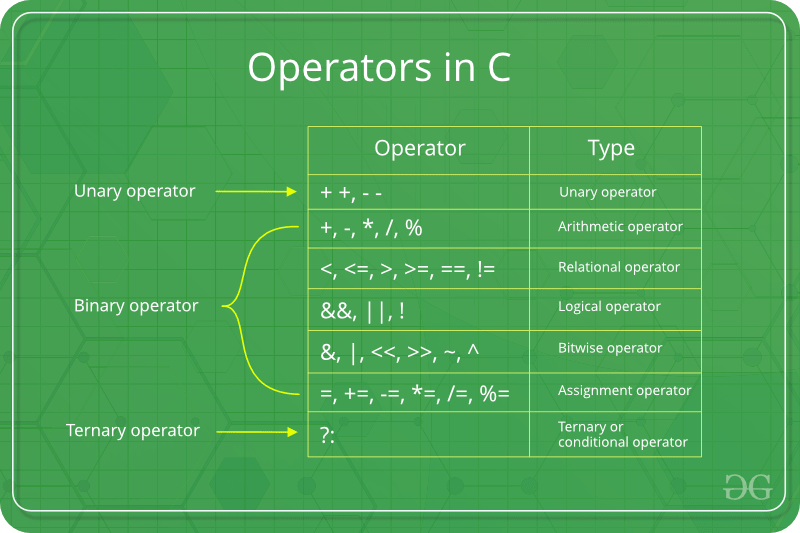# Assignment Operators in C/C++Assignment operators are used to assigning value to a variable. The left side operand of the assignment operator is a variable and right side operand of the assignment operator is a value. The value on the right side must be of the same data-type of the variable on the left side otherwise the compiler will raise an error.
Different types of assignment operators are shown below:

• “=”: This is the simplest assignment operator. This operator is used to assign the value on the right to the variable on the left.
For example:

```a = 10;
b = 20;
ch = 'y';
```
• “+=”: This operator is combination of ‘+’ and ‘=’ operators. This operator first adds the current value of the variable on left to the value on the right and then assigns the result to the variable on the left.
Example:

```(a += b) can be written as (a = a + b)
```

If initially value stored in a is 5. Then (a += 6) = 11.

• “-=”This operator is combination of ‘-‘ and ‘=’ operators. This operator first subtracts the current value of the variable on left from the value on the right and then assigns the result to the variable on the left.
Example:

```(a -= b) can be written as (a = a - b)
```

If initially value stored in a is 8. Then (a -= 6) = 2.

• “*=”This operator is combination of ‘*’ and ‘=’ operators. This operator first multiplies the current value of the variable on left to the value on the right and then assigns the result to the variable on the left.
Example:

```(a *= b) can be written as (a = a * b)
```

If initially value stored in a is 5. Then (a *= 6) = 30.

• “/=”This operator is combination of ‘/’ and ‘=’ operators. This operator first divides the current value of the variable on left by the value on the right and then assigns the result to the variable on the left.
Example:

```(a /= b) can be written as (a = a / b)
```

If initially value stored in a is 6. Then (a /= 2) = 3.

Below example illustrates the various Assignment Operators:

## C

 `// C program to demonstrate ` `// working of Assignment operators ` ` `  `#include ` ` `  `int` `main() ` `{ ` ` `  `    ``// Assigning value 10 to a ` `    ``// using "=" operator ` `    ``int` `a = 10; ` `    ``printf``(``"Value of a is %d\n"``, a); ` ` `  `    ``// Assigning value by adding 10 to a ` `    ``// using "+=" operator ` `    ``a += 10; ` `    ``printf``(``"Value of a is %d\n"``, a); ` ` `  `    ``// Assigning value by subtracting 10 from a ` `    ``// using "-=" operator ` `    ``a -= 10; ` `    ``printf``(``"Value of a is %d\n"``, a); ` ` `  `    ``// Assigning value by multiplying 10 to a ` `    ``// using "*=" operator ` `    ``a *= 10; ` `    ``printf``(``"Value of a is %d\n"``, a); ` ` `  `    ``// Assigning value by dividing 10 from a ` `    ``// using "/=" operator ` `    ``a /= 10; ` `    ``printf``(``"Value of a is %d\n"``, a); ` ` `  `    ``return` `0; ` `} `

## C++

 `// C++ program to demonstrate  ` `// working of Assignment operators  ` ` `  `#include ` `using` `namespace` `std; ` ` `  `int` `main()  ` `{  ` ` `  `    ``// Assigning value 10 to a  ` `    ``// using "=" operator  ` `    ``int` `a = 10;  ` `    ``cout << ``"Value of a is "``<

Output:

```Value of a is 10
Value of a is 20
Value of a is 10
Value of a is 100
Value of a is 10
```

My Personal Notes arrow_drop_upCheck out this Author's contributed articles.

If you like GeeksforGeeks and would like to contribute, you can also write an article using contribute.geeksforgeeks.org or mail your article to contribute@geeksforgeeks.org. See your article appearing on the GeeksforGeeks main page and help other Geeks.

Please Improve this article if you find anything incorrect by clicking on the "Improve Article" button below.

Article Tags :
Practice Tags :

3

Please write to us at contribute@geeksforgeeks.org to report any issue with the above content.